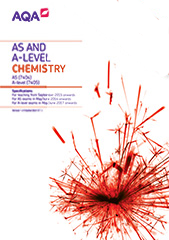# 3.1.9 Rate equations (A-level only)

In rate equations, the mathematical relationship between rate of reaction and concentration gives information about the mechanism of a reaction that may occur in several steps.

## Rate equations (A-level only)

Content

Opportunities for skills development

The rate of a chemical reaction is related to the concentration of reactants by a rate equation of the form:

Rate = k[A]m [B]n

where m and n are the orders of reaction with respect to reactants A and B and k is the rate constant.

The orders m and n are restricted to the values 0, 1, and 2.

The rate constant k varies with temperature as shown by the equation:

k = AeEa/RT

where A is a constant, known as the Arrhenius constant, Ea is the activation energy and T is the temperature in K.

Students should be able to:

• define the terms order of reaction and rate constant
• perform calculations using the rate equation
• explain the qualitative effect of changes in temperature on the rate constant k
• perform calculations using the equation k = Ae–Ea/RT
• understand that the equation k = Ae–Ea/RT can be rearranged into the form ln k = –Ea /RT + ln A and know how to use this rearranged equation with experimental data to plot a straight line graph with slope –Ea/R

These equations and the gas constant, R, will be given when required.

MS 0.0 and 2.4

Students use given rate data and deduce a rate equation, then use some of the data to calculate the rate constant including units. Rate equations could be given and students asked to calculate rate constant or rate.

MS 3.3 and 3.4

Students use a graph of concentration–time and calculate the rate constant of a zero-order reaction by determination of the gradient.

## Determination of rate equation (A-level only)

Content

Opportunities for skills development

The rate equation is an experimentally determined relationship.

The orders with respect to reactants can provide information about the mechanism of a reaction.

Students should be able to:

• use concentration–time graphs to deduce the rate of a reaction
• use initial concentration–time data to deduce the initial rate of a reaction
• use rate–concentration data or graphs to deduce the order (0, 1 or 2) with respect to a reactant
• derive the rate equation for a reaction from the orders with respect to each of the reactants
• use the orders with respect to reactants to provide information about the rate determining/limiting step of a reaction.

AT a, b, k and l

PS 2.4 and 3.1

Students could determine the order of reaction for a reactant in the iodine clock reaction.

MS 3.1

Students could be given data to plot and interpret in terms of order with respect to a reactant. Alternatively, students could just be given appropriate graphs and asked to derive order(s).

MS 3.3 and 3.4

Students calculate the rate constant of a zero-order reaction by determining the gradient of a concentration–time graph.

MS 3.5

Students plot concentration–time graphs from collected or supplied data and draw an appropriate best-fit curve.

Students draw tangents to such curves to deduce rates at different times.

Required practical 7Measuring the rate of reaction:
• by an initial rate method
• by a continuous monitoring method.